Q-Chem 5.0 User’s Manual

# A.2 Theoretical Background

Consider the energy,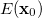at some point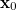on a potential energy surface. We can express the energy at a nearby point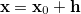by means of the Taylor series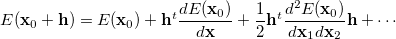(A.1)

If we knew the exact form of the energy functional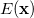and all its derivatives, we could move from the current pointdirectly to a stationary point, (i.e., we would know exactly what the step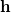ought to be). Since we typically know only the lower derivatives ofat best, then we can estimate the stepby differentiating the Taylor series with respect to, keeping only the first few terms on the right hand side, and setting the left hand side,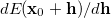, to zero, which is the value it would have at a genuine stationary point. Thus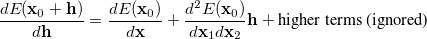(A.2)

from which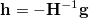(A.3)

where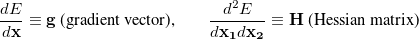(A.4)

Equation (A.3) is known as the Newton-Raphson step. It is the major component of almost all geometry optimization algorithms in quantum chemistry.

The above derivation assumed exact first (gradient) and second (Hessian) derivative information. Analytical gradients are available for all methodologies supported in Q-Chem; however analytical second derivatives are not. Furthermore, even if they were, it would not necessarily be advantageous to use them as their evaluation is usually computationally demanding, and, efficient optimizations can in fact be performed without an exact Hessian. An excellent compromise in practice is to begin with an approximate Hessian matrix, and update this using gradient and displacement information generated as the optimization progresses. In this way the starting Hessian can be “improved” at essentially no cost. Using Eq. (A.3) with an approximate Hessian is called the quasi Newton-Raphson step.

The nature of the Hessian matrix (in particular its eigenvalue structure) plays a crucial role in a successful optimization. All stationary points on a potential energy surface have a zero gradient vector; however the character of the stationary point (i.e., what type of structure it corresponds to) is determined by the Hessian. Diagonalization of the Hessian matrix can be considered to define a set of mutually orthogonal directions on the energy surface (the eigenvectors) together with the curvature along those directions (the eigenvalues). At a local minimum (corresponding to a well in the potential energy surface) the curvature along all of these directions must be positive, reflecting the fact that a small displacement along any of these directions causes the energy to rise. At a transition state, the curvature is negative (i.e., the energy is a maximum) along one direction, but positive along all the others. Thus, for a stationary point to be a transition state the Hessian matrix at that point must have one and only one negative eigenvalue, while for a minimum the Hessian must have all positive eigenvalues. In the latter case the Hessian is called positive definite. If searching for a minimum it is important that the Hessian matrix be positive definite; in fact, unless the Hessian is positive definite there is no guarantee that the step predicted by Eq. (A.3) is even a descent step (i.e., a direction that will actually lower the energy). Similarly, for a transition state search, the Hessian must have one negative eigenvalue. Maintaining the Hessian eigenvalue structure is not difficult for minimization, but it can be a difficulty when trying to find a transition state.

In a diagonal Hessian representation the Newton-Raphson step can be written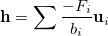(A.5)

where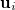and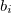are the eigenvectors and eigenvalues of the Hessian matrix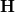and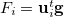is the component of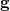along the local direction (eigenmode). As discussed by Simons et al. , the Newton-Raphson step can be considered as minimizing along directionswhich have positive eigenvalues and maximizing along directions with negative eigenvalues. Thus, if the user is searching for a minimum and the Hessian matrix is positive definite, then the Newton-Raphson step is appropriate since it is attempting to minimize along all directions simultaneously. However, if the Hessian has one or more negative eigenvalues, then the basic Newton-Raphson step is not appropriate for a minimum search, since it will be maximizing and not minimizing along one or more directions. Exactly the same arguments apply during a transition state search except that the Hessian must have one negative eigenvalue, because the user has to maximize along one direction. However, there must be only one negative eigenvalue. A positive definite Hessian is a disaster for a transition state search because the Newton-Raphson step will then lead towards a minimum.

If firmly in a region of the potential energy surface with the right Hessian character, then a careful search (based on the Newton-Raphson step) will almost always lead to a stationary point of the correct type. However, this is only true if the Hessian is exact. If an approximate Hessian is being improved by updating, then there is no guarantee that the Hessian eigenvalue structure will be retained from one cycle to the next unless one is very careful during the update. Updating procedures that “guarantee” conservation of a positive definite Hessian do exist (or at least warn the user if the update is likely to introduce negative eigenvalues). This can be very useful during a minimum search; but there are no such guarantees for preserving the Hessian character (one and only one negative eigenvalue) required for a transition state.

In addition to the difficulties in retaining the correct Hessian character, there is the matter of obtaining a “correct” Hessian in the first instance. This is particularly acute for a transition state search. For a minimum search it is possible to “guess” a reasonable, positive-definite starting Hessian (for example, by carrying out a molecular mechanics minimization initially and using the mechanics Hessian to begin the ab initio optimization) but this option is usually not available for transition states. Even if the user calculates the Hessian exactly at the starting geometry, the guess for the structure may not be sufficiently accurate, and the expensive, exact Hessian may not have the desired eigenvalue structure.

Consequently, particularly for a transition state search, an alternative to the basic Newton-Raphson step is clearly needed, especially when the Hessian matrix is inappropriate for the stationary point being sought.

One of the first algorithms that was capable of taking corrective action during a transition state search if the Hessian had the wrong eigenvalue structure, was developed by Poppinger , who suggested that, instead of taking the Newton-Raphson step, if the Hessian had all positive eigenvalues, the lowest Hessian mode be followed uphill; whereas, if there were two or more negative eigenvalues, the mode corresponding to the least negative eigenvalue be followed downhill. While this step should lead the user back into the right region of the energy surface, it has the disadvantage that the user is maximizing or minimizing along one mode only, unlike the Newton-Raphson step which maximizes/minimizes along all modes simultaneously. Another drawback is that successive such steps tend to become linearly dependent, which degrades most of the commonly used Hessian updates.